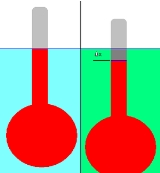xRelative densityOverview

Relative density, or specific gravity, is the ratio
Ratio
In mathematics, a ratio is a relationship between two numbers of the same kind , usually expressed as "a to b" or a:b, sometimes expressed arithmetically as a dimensionless quotient of the two which explicitly indicates how many times the first number contains the second In mathematics, a ratio is...

of the density
Density
The mass density or density of a material is defined as its mass per unit volume. The symbol most often used for density is ρ . In some cases , density is also defined as its weight per unit volume; although, this quantity is more properly called specific weight...

(mass of a unit volume) of a substance to the density of a given reference material. Specific gravity usually means relative density with respect to water. The term "relative density" is often preferred in modern scientific usage.

If a substance's relative density is less than one then it is less dense than the reference; if greater than 1 then it is denser than the reference.